Courses

# NCERT Solutions Chapter 7 - Congruence of Triangles, Maths, Class 7 | EduRev Notes

## Class 7 : NCERT Solutions Chapter 7 - Congruence of Triangles, Maths, Class 7 | EduRev Notes

The document NCERT Solutions Chapter 7 - Congruence of Triangles, Maths, Class 7 | EduRev Notes is a part of the Class 7 Course Mathematics (Maths) Class 7.
All you need of Class 7 at this link: Class 7

Exercise 7.1

Question 1:

Complete the following statements:

(a) Two line segments are congruent if _______________.

(b) Among two congruent angles, one has a measure of 70o, the measure of other angle is _______________.

(c) When we write ∠A = ∠B, we actually mean _______________.

Answer 1:

(a) they have the same length

(b) 70o

(c) mA = mB

Question 2:

Give any two real time examples for congruent shapes.

Answer 2:

(i) Two footballs

(ii) Two teacher’s tables

Question 3:

If ΔABC ≌  ΔFED under the correspondence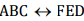, write all the corresponding congruent parts of the triangles.

Answer 3: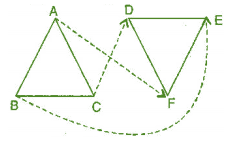Given: ΔABC ≌  ΔFED.

The corresponding congruent parts of die triangles are: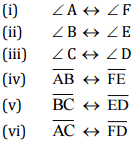Question 4:

If ΔDEF ≌  ΔBCA, write the part (s) of ΔBCA that correspond to: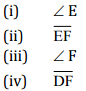Answer 4: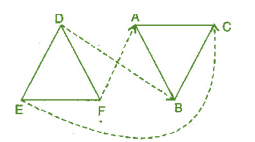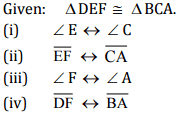Exercise 7.2

Question 1:

Which congruence criterion do you use in the following?

(a) Given:    AC = DF, AB = DE, BC = EF
So    ΔABC ≌  ΔDEF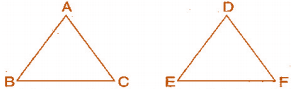(b) Given:    RP = ZX, RQ = ZY, ∠PRQ = ∠XZY
So    ΔPQR ≌  ΔXYZ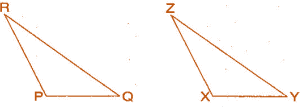(c) Given:    ∠ MLN = ∠ FGH, ∠ NML = ∠ HFG, ML = FG
So    ΔLMN ≌ ΔGFH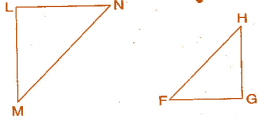(d) Given:    EB = BD, AE = CB, ∠A = ∠C = 90°
So    AABE = ACDB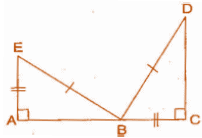Answer 1:

(a) By SSS congruence criterion,  since it is given that AC = DF, AB = DE, BC = EF
The three sides of one triangle are equal to the three corresponding sides of another triangle.
Therefore, ΔABC ≌  ΔDEF

(b) By SAS congruence criterion,  since it is given that RP = ZX, RQ = ZY and ∠PRQ = ∠XZY
The two sides and one angle in one of the triangle are equal to the corresponding sides and the angle of other triangle.
Therefore, ΔPQR ≌ ΔXYZ

(c) By ASA congruence criterion, since it is given that ∠MLN = ∠FGH, ∠NML = ∠HFG, ML = FG.
The two angles and one side in one of the triangle are equal to the corresponding angles and side of other triangle.
Therefore, ΔLMN ≌  ΔGFH

(d) By RHS congruence criterion, since it is given that EB = BD, AE = CB, ∠A = ∠C = 90°
Hypotenuse and one side of a right angled triangle are respectively equal to the hypotenuse and one side of another right angled triangle.
Therefore, ΔABE ≌  ΔCDB

Question 2:

You want to show that ΔART ≌  ΔPEN:
(a) If you have to use SSS criterion, then you need to show:
(i) AR =
(ii) RT =
(iii) AT =

(b) If it is given that ∠T = ∠N and you are to use SAS criterion, you need to have:
(i) RT =    and
(ii) PN =

(c) If it is given that AT = PN and you are to use ASA criterion, you need to have:
(i) ?
(ii) ?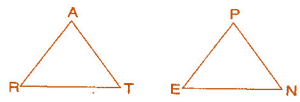Answer 2:

(a) Using SSS criterion,    ΔART ≌  ΔPEN
(i) AR = PE
(ii) RT= EN
(iii) AT = PN

(b) Given: ∠T = ∠N
Using SAS criterion,    ΔART ≌  ΔPEN
(i) RT = EN
(ii) PN = AT

(c) Given:  AT = PN
Using ASA criterion,    ΔART ≌  ΔPEN
(i) ∠RAT = ∠EPN
(ii) ∠RTA = ∠ENP

Question 3:

You have to show that ΔAMP = ΔAMQ. In the following proof supply the missing reasons: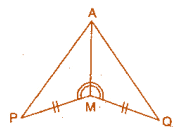Steps Reasons (i) PM = QM (i) (ii) ∠ PMA = ∠ QMA (ii) (iii) AM = AM (iii) (iv) ΔAMP ≌ ΔAMQ (iv)

Answer 3:

 Steps Reasons (i) PM = QM (i) Given (ii) ∠PMA = ∠QMA (ii) Given (iii) AM = AM (iii) Common (iv) Δ AMP ≌ ΔAMQ (iv) SAS congruence rule

Question 4:

In ΔABC, ∠A = 30° ∠B = 40° and ∠C = 110°
In ΔPQR, ∠P = 30° ∠Q = 40° and ∠R = 110°.
A student says that ΔABC ≌ ΔPQR by AAA congruence criterion. Is he justified? Why or why not?

Answer 4:

No, because the two triangles with equal corresponding angles need not be congruent. In such a correspondence, one of them can be an enlarged copy of the other.

Question 5:

In the figure, the two triangles are congruent. The corresponding parts are marked. We can write Δ RAT ≌ ?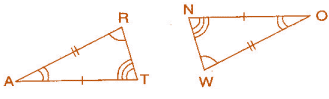Answer 5:

In the figure, given two triangles are congruent. So, the corresponding parts are: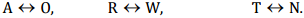We can write, ΔRAT ≌ ΔWON    [By SAS congruence rule]

Question 6:

Complete the congruence statement: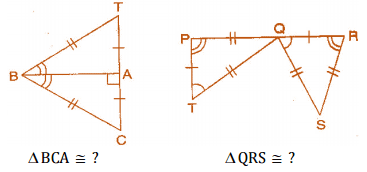Answer 6:

In A BAT and ABAC, given triangles are congruent so the corresponding parts are: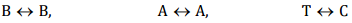Thus, ΔBCA ≌ ΔBTA    |By SSS congruence rule]
In ΔQRS and ΔTPQ, given triangles are congruent so the corresponding parts are: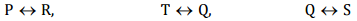Thus, ΔQRS ≌  ΔTPQ    [By SSS congruence rule]

Question 7:

In a squared sheet, draw two triangles of equal area such that:

(i) the triangles are congruent.

(ii) the triangles are not congruent.

What can you say about their perimeters?

Answer 7:

In a squared sheet, draw ΔABC and ΔPQR. When two triangles have equal areas and

(i)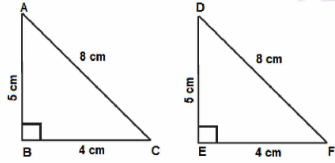In the above figure, ΔABC and ΔDEF have equal areas.
And also,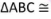ΔDEF
So, we can say that perimeters of ΔABC and ΔDEF are equal.

(ii)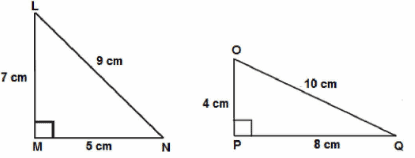In the above figure, ΔLMN and ΔOPQ
ΔLMN is not congruent to ΔOPQ
So, we can also say that their perimeters are not same.

Question 8:

Draw a rough sketch of two triangles such that they have five pairs of congruent parts but still the triangles are not congruent.

Answer 8:

Let us draw two triangles PQR and ABC.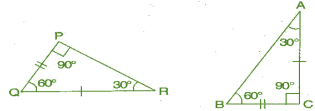All angles are equal, two sides are equal except one side. Hence, ΔPQR are not congruent to ΔABC.

Question 9:

If ΔABC and ΔPQR are to be congruent, name one additional pair of corresponding parts. What criterion did you use?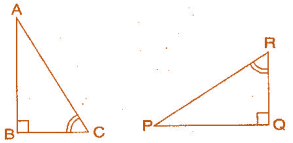Answer 9:

A ABC and A PQR are congruent Then one additional pair is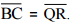Given: ∠B = ∠Q = 90°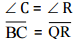Therefore, ΔABC ≌ ΔPQR    [By ASA congruence rule]

Question 10:

Explain, why ΔABC  ΔFED.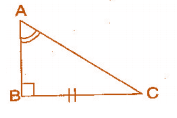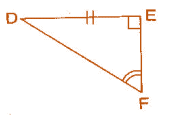Answer 10:

Given:    ∠A = ∠F, BC = ED, ∠B = ∠E
In ΔABC and ΔFED,
∠B = ∠E = 90°
∠A = ∠F
BC = ED
Therefore, ΔABC ≌ ΔFED    [By RHS congruence rule]

Offer running on EduRev: Apply code STAYHOME200 to get INR 200 off on our premium plan EduRev Infinity!

## Mathematics (Maths) Class 7

209 videos|109 docs|45 tests

,

,

,

,

,

,

,

,

,

,

,

,

,

,

,

,

,

,

,

,

,

,

,

,

,

,

,

;